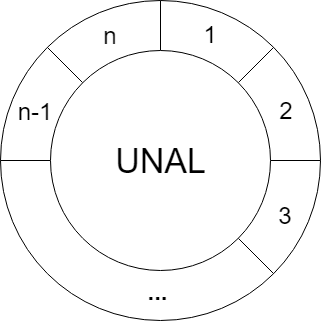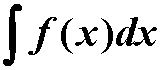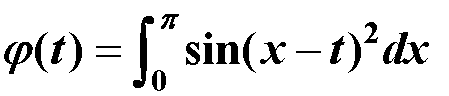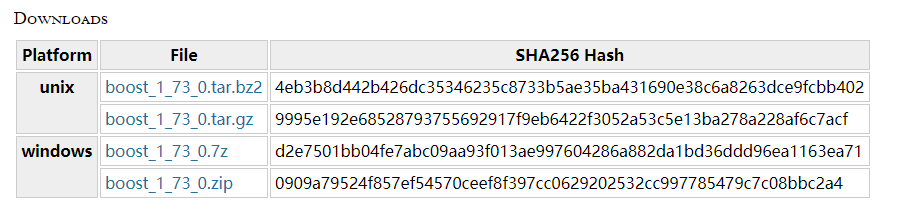# 2019徐州网络赛 I query 线段树 离线

``````#include <bits/stdc++.h>
using namespace std;
const int N = 1e5 + 10;
int pos[N];
int a[N];
int n, m;
vector<int> v[N];
struct node {int l, r;int val;
}tree[N << 2];
struct QUE {int l, r;int id;
}q[N];
int ans[N];
bool cmp1(QUE x, QUE y) {return x.r < y.r;
}
void build(int l, int r, int cur) {tree[cur].l = l;tree[cur].r = r;tree[cur].val = 0;if(l == r) return;int mid = (l + r) >> 1;build(l, mid, cur << 1);build(mid + 1, r, cur << 1 |1);
}
void update(int pos, int cur) {if(tree[cur].l == tree[cur].r) {tree[cur].val++;return;}if(pos <= tree[cur << 1].r) update(pos, cur <<1);else update(pos, cur << 1 |1);tree[cur].val = tree[cur << 1].val + tree[cur << 1 | 1].val;
}
int query(int pl, int pr, int cur) {if(pl <= tree[cur].l && tree[cur].r <= pr) {return tree[cur].val;}int res = 0;if(pl <= tree[cur << 1].r) res += query(pl, pr, cur << 1);if(pr >= tree[cur << 1 | 1].l) res += query(pl, pr, cur << 1 | 1);return res;
}
int main() {scanf("%d %d", &n, &m);for(int i = 1; i <= n; i++) {scanf("%d", &a[i]);pos[a[i]] = i;}int l, r;for(int i = 1; i <= n; i++) {for(int j = a[i] + a[i]; j <= n; j += a[i]) {l = i;r = pos[j];if(l > r) swap(l, r);v[r].push_back(l);}}for(int i = 1; i <= m; i++)scanf("%d %d", &q[i].l, &q[i].r), q[i].id = i;sort(q + 1, q+ 1 + m, cmp1);build(1, n, 1);for(int j = 1, i = 1; i <= n; i++) {for(int k = 0; k < v[i].size(); k++)update(v[i][k], 1);while(j <= m && q[j].r == i) {ans[q[j].id] = query(q[j].l, q[j].r, 1);j++;}}for(int i = 1; i <= m; i++)printf("%d\n", ans[i]);return 0;
}``````

### 相关文章：## 2019徐州网络赛 I query 线段树 离线## 2019徐州网络赛 J、Random Access Iterator 树上 概率 dp## 2019银川网络赛A？ FZU - 2105 Digits Count 线段树 区间操作 | ^## 2019 徐州网络赛 F. Little M's attack plan 树上倍增+dfs序+二分## HDU - 5489 Removed Interval LIS+枚举技巧## 2019 南昌网络赛 C Hello 2019 / CodeForces - 750ENew Year and Old Subsequence 线段树维护矩阵## 构造螺旋矩阵 n*m## 2019 上海网络赛 B、 Light bulbs 区间差分 / 线段树点维护区间## 2019 上海网络赛 G、Substring 字符串哈希## 2019 沈阳网络赛 H. Texas hold'em Poker 模拟## 2019 沈阳网络赛 B. Dudu's maze dfs 联通块选取## 51nod 1571 最近等对 CQD分治## 牛客 Fruit Ninja 随机大法## HDU - 6242 Geometry Problem 随机数## HDU - 6393 Traffic Network in Numazu 树上倍增求lca+dfs序+线段树## CodeForces - 1220D Alex and Julian 二分图性质## CodeForces - 1220E Tourism 无向图缩点+树形dp## HDU - 6156 Palindrome Function 数位dp## HDU - 6153 A Secret 扩展kmp## Gym - 100589A Queries on the Tree 树状数组+分块## HDU-6731 Angle Beats 计算几何 直角三角形个数## HDU - 5927 Auxiliary Set dfs序+瞎搞## CodeForces - 689D Friends and Subsequences ST表+二分## 2017南宁 I. Rake It In dfs博弈## CodeForces - 369E Valera and Queries 树状数组+离线+容斥思想## Gym - 101889I Imperial roads 树链剖分+最小生成树之必选一边## CodeForces - 1228C Primes and Multiplication 数贡献## CodeForces - 1228D Complete Tripartite 图上的哈希## 2017 ICPC Asia Urumqi I. A Possible Tree 带权并查集## HDU - 5923 Prediction 多个并查集## 2018徐州 Gym - 102028J Carpets Removal 概率事件+二维差分## Gym - 102307J Jail Destruction 线段树## Gym - 101955L Machining Disc Rotors 计算几何 圆剩余部分直径## Gym - 102307E Extreme Image 线段树 扫描线## Gym - 102307C Common Subsequence dp## 18南京 Gym - 101981M Mediocre String Problem 扩展kmp+马拉车## CodeForces - 374D Inna and Sequence 线段树## ICPC Asia HongKong 2017## 1225 D. Power Products 哈希## 1080 F. Katya and Segments Sets 主席树## Kattis - largesttriangle Largest Triangle 平面最大三角形面积## 数据挖掘-k平均算法

/* 题目内容&#xff1a;实现k平均算法的代码&#xff0c;并且通过样例 代码&#xff1a; */ #include <bits/stdc.h> using namespace std; #define eps 1e-8 #define INF 0x3f3f3f3f const int N 1e6 10; int n, k; pair<double, double> a[N], b[N]; vector&l…...## 初识matlab## MATLAB学习知识整理 从入门到放弃## matlab数组运算与数组化编程

matlab中的运算和操作主要是以数组为对象的&#xff0c; 数组又包括&#xff1a;数值数组、字符数组、元胞数组等。 一、数值数组的建立&#xff1a; 1. 直接输入法&#xff1a; 逗号&#xff1a;用来分开数组中的行元素。&#xff08;可用空格代替&#xff09; 分号&#…...## matlab程序设计## matlab数值计算## matlab图形功能

//...## python实现键盘输入多个值

Python 2里面读取输入的函数是raw_input()&#xff0c; Python 3的是input()&#xff0c;读入一个值后回车读取输入就退出了&#xff0c;想要一次读取多个输入&#xff0c;可以像下面这样&#xff1a; a, b raw_input().split()1 2a Out: 1b Out: 2 上面保存的是字…...